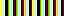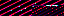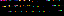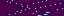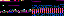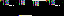```<?php

function include_configuration_file(\$file_path, \$languages="all")
{
if (!file_exists(\$file_path)) return;

\$content = file(\$file_path);
\$operations = array("define_in_php", "write_to_javascript_file");

if (\$languages == "all" || \$languages == "javascript")
{
erase_file_contents(JAVASCRIPT_CONFIG_FILE_PATH);
}

switch (\$languages)
{
case "php":
return parse_configuration(\$content, \$operations);
case "javascript":
return parse_configuration(\$content, \$operations);
default:
return parse_configuration(\$content, \$operations);
}
}

function parse_configuration(\$body, \$operations=NULL)
{
\$operations = (!is_array(\$operations)) ? array(\$operations) : \$operations;
foreach (\$body as \$line)
{
if (preg_match("/^ *#.*\$/", \$line) || trim(\$line) == "") continue;
\$equality = explode("=", \$line, 2);
\$variable = strtoupper(trim(\$equality));
\$variable = str_replace(" ", "_", \$variable);
\$value = trim(\$equality);
foreach (\$operations as \$operation)
{
call_user_func(\$operation, \$variable, \$value);
}
}
}

function write_to_javascript_file(\$variable, \$value)
{
\$equality = "var " . \$variable . " = \"" . \$value . "\";\n";
file_put_contents(JAVASCRIPT_CONFIG_FILE_PATH, \$equality, FILE_APPEND);
}

function define_in_php(\$variable, \$value)
{
\$GLOBALS[\$variable] = \$value;
}

function erase_file_contents(\$file_path)
{
\$fp = fopen(\$file_path, 'w');
fclose(\$fp);
}
```
```<?php
namespace type;

function convert_to_array(\$item)
{
if (is_array(\$item) == False)
{
return array(\$item);
}

return \$item;
}
```
```<?php
namespace sort;

function quick_sort(array &\$array, \$comparer=null, \$left=0, \$right=null)
{
\$right = set_right_index(\$right, \$array);
if (\$right > \$left)
{
\$pivot = calculate_pivot_index(\$left, \$right);
\$new_pivot = partition(\$array, \$comparer, \$left, \$right, \$pivot);
quick_sort(\$array, \$comparer, \$left, \$new_pivot - 1);
quick_sort(\$array, \$comparer, \$new_pivot + 1, \$right);
}
}

function set_right_index(\$index, \$array)
{
if (is_null(\$index))
{
\$index = count(\$array) - 1;
}
return \$index;
}

function calculate_pivot_index(\$left_index, \$right_index)
{
\$pivot_index = \$right_index - (\$right_index - \$left_index) / 2;
return \$pivot_index;
}

function partition(&\$array, \$comparer, \$left, \$right, \$pivot_index)
{
\$pivot_value = \$array[\$pivot_index];
swap(\$array, \$right, \$pivot_index);
\$current = \$left;
for (\$ii = \$left; \$ii < \$right; \$ii++)
{
\$comparison = make_comparison(\$comparer, \$array[\$ii], \$pivot_value);
if (\$comparison === True)
{
swap(\$array, \$ii, \$current++);
}
}
swap(\$array, \$current, \$right);
return \$current;
}

function swap(&\$array, \$index_1, \$index_2)
{
\$value = \$array[\$index_1];
\$array[\$index_1] = \$array[\$index_2];
\$array[\$index_2] = \$value;
}

function make_comparison(\$comparer, \$value_1, \$value_2)
{
if (!is_null(\$comparer))
{
\$comparison = call_user_func(\$comparer, \$value_1, \$value_2);
}
else
{
\$comparison = (\$value_1 < \$value_2);
}
return \$comparison;
}
```
```<?php
namespace file;

function count_depth(\$path)
{
\$stripped_path = trim(\$path, "/");
return sizeof(explode("/", \$path));
}
```
```<?php

function remove_directory(\$directory_path, \$remove_self=True)
{
\$directory_path = rtrim(\$directory_path, "/") . "/";
\$directory = opendir(\$directory_path);
{
if (\$file == '.') continue;

\$relative_path = \$directory_path . \$file;
if (is_dir(\$relative_path))
{
remove_directory(\$relative_path);
continue;
}
}
if (\$remove_self) rmdir(\$directory_path);
}
```
```<?php
namespace file;

function is_directory_empty(\$directory_path)
{
\$files = scandir(\$directory_path);
return count(\$files) > 2;
}
```
```<?php

function print_file_range(\$fp, \$start, \$end)
{
fseek(\$fp, \$start);
echo "\$start - \$end\n";

}
```
```<?php

function build_path(\$directory_path, \$file_path)
{
\$directory_path = rtrim(\$directory_path, "/") . "/";
\$path = \$directory_path . \$file_path;
return \$path;
}
```
44.200.117.166
44.200.117.166
44.200.117.166

July 19, 2017

f1. BOSS

Games are corrupt dissolutions of nature modeled on prison, ordering a census from the shadows of a vile casino, splintered into shattered glass, pushing symbols, rusted, stale, charred, ultraviolet harbingers of consumption and violence, badges without merit that host a disease of destruction and decay.

You are trapped. You are so trapped your only recourse of action is to imagine an escape route and deny your existence so fully that your dream world becomes the only reality you know. You are fleeing deeper and deeper into a chasm of self-delusion.

While you're dragging your listless, distending corpus from one cell to another, amassing rewards, upgrades, bonuses, achievements, prizes, add-ons and status boosts in rapid succession, stop to think about what's inside the boxes because each one contains a vacuous, soul-sucking nightmare.

Playing can be an awful experience that spirals one into a void of harm and chaos, one so bad it creates a cycle between the greater and lesser systems, each breaking the other's rules. One may succeed by acting in a way that ruins the world.